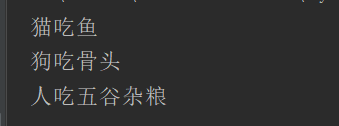5G时代下一个聚合的编程学习网

# python的继承、封装、多态 –面向对象的特征

python同样可有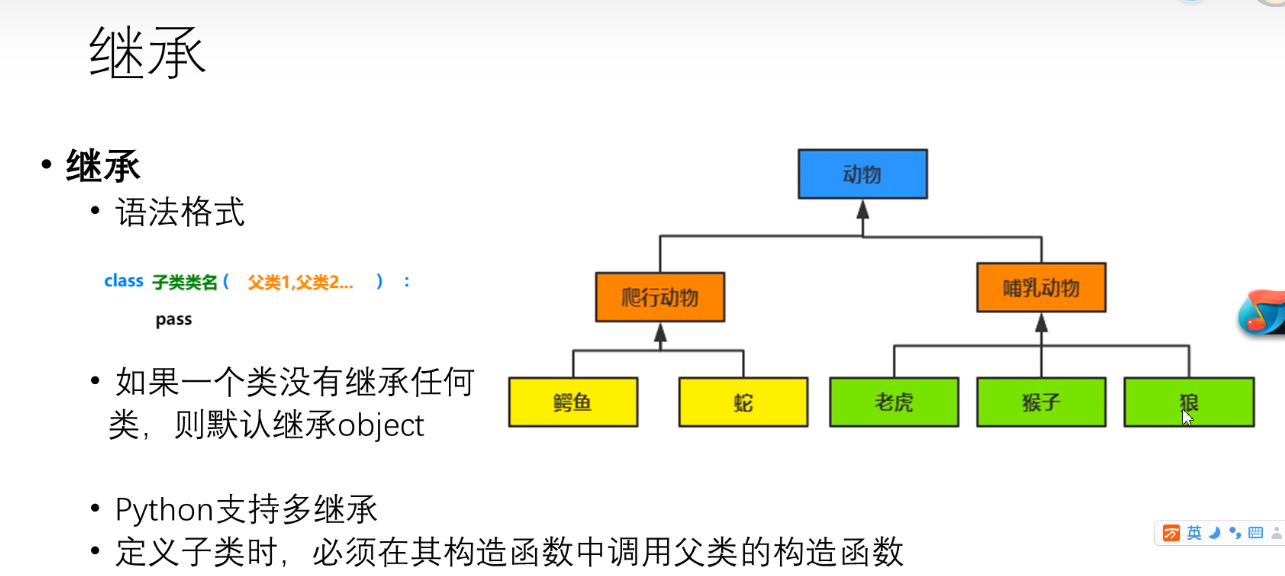```class Person():
def __init__(self,name,age):
self.name =name
self.age=age
def info(self):
print(self.name,self.age)

class Thomas(Person):
def __init__(self,name,age,score):
super().__init__(name,age)
self.score=score

stu=Thomas('jack',20,'100')
stu.info()
```

输出的结果为：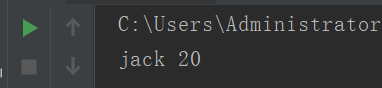（2）一个类继承多个父类

```class A():
def a(self):
print('方法A')
class B():
def b(self):
print('方法B')
class C(A,B):
def __init__(self,name):
print('方法C')
d=C('a')
d.a()
d.b()
```

输出结果：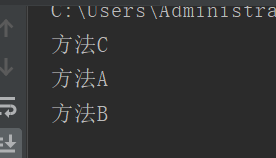（3）方法重写

```class Person():
def __init__(self,name,age):
self.name =name
self.age=age
def info(self):
print(self.name,self.age)

class Thomas(Person):
def __init__(self,name,age,score):
super().__init__(name,age)
self.score=score
def info(self):
print(self.score)
super().info()
stu=Thomas('jack',20,'100')
stu.info()
```

12行是对父类方法info的重写

13是增加自己想输出的内容

14行是输出继承父类的内容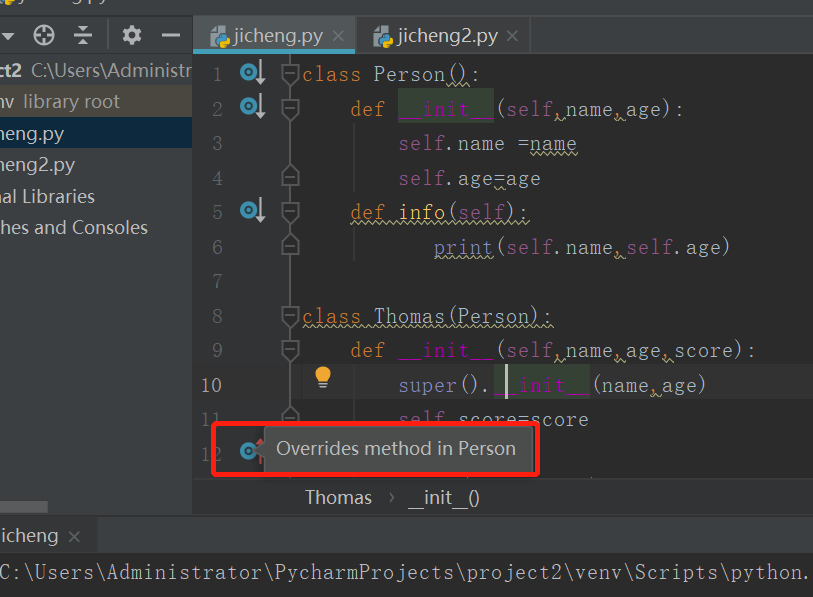在info方法的左侧有个重写的提示

—————————-

object类

object:类是所有类的父关，因此所有类都有oject类的属性和方法内置函数dir()可以查看指定对象所有属性

Object有一个_str_()方法，用于返回一个对于“对象的描述”对应于内置函数str()经常用于 print()方法，帮我们查看对象的信息，所以我们经常会对_str_()进行重写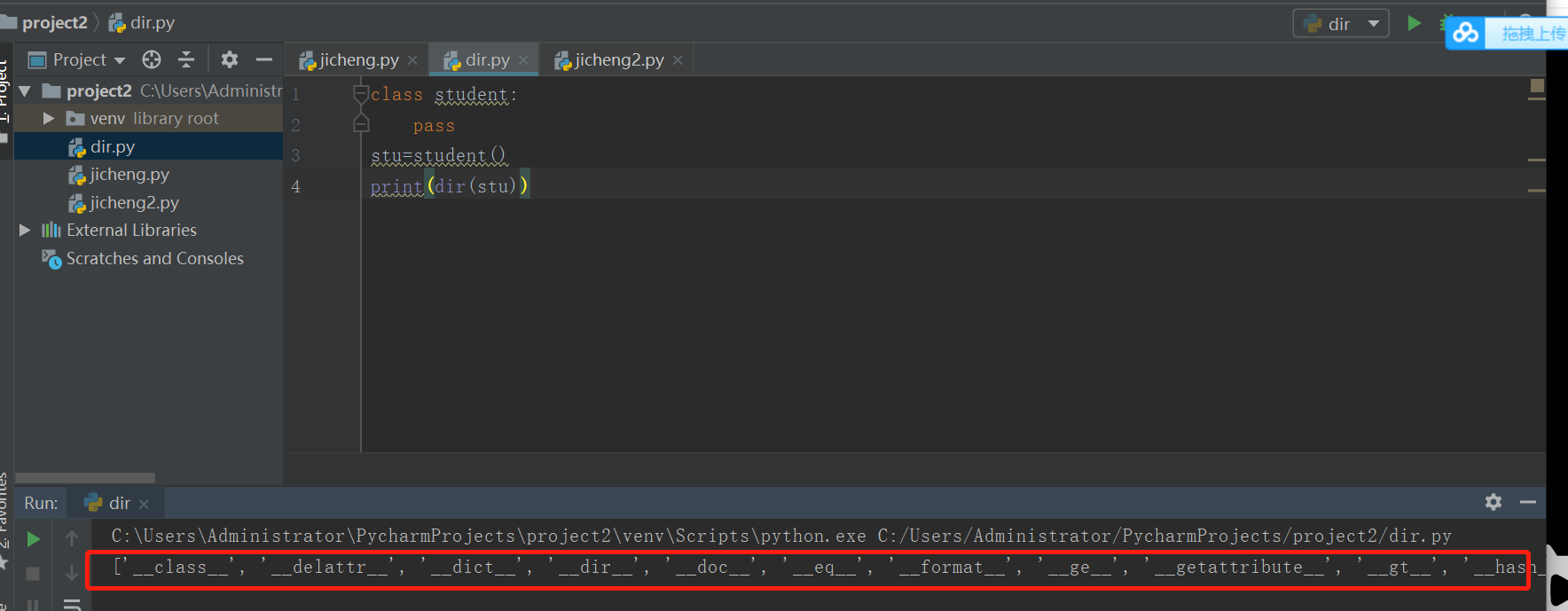```class Animal(object):
def eat(self):
print('动物会吃')

class  Dog(Animal):
def eat(self):
print('狗吃骨头')

class Cat(Animal):
def eat(self):
print('猫吃鱼')

class Person():
def eat(self):
print('人吃五谷杂粮')

def fun(obj):
obj.eat()

fun(Cat())
fun(Dog())
fun(Person())
```

输出的结果为：